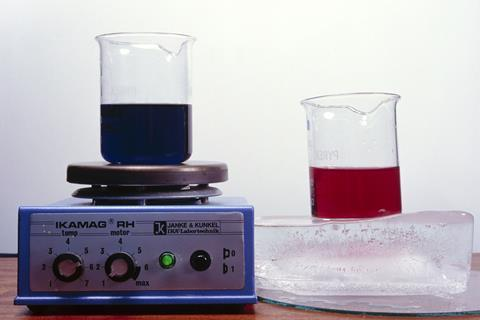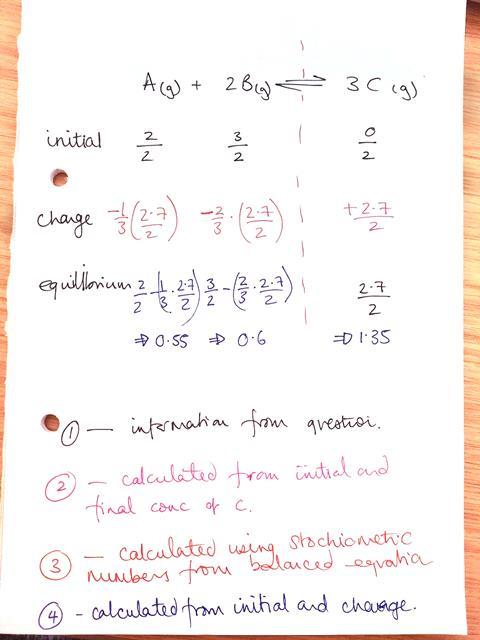Support your post-16 students in their understanding of equilibriumUse the concept of someone walking up an escalator as it moves down to help chemistry students grasp equilibrium

## Context setting

There are several classic equilibrium reactions that we talk about in chemistry, like the Haber process and the contact process, which are fundamental to the global economy. The Haber process produces around 240 million tonnes of ammonia a year (this was the case in 2020) which is mostly used to increase crop production to support the food needs of the planet’s population. But getting good yields of ammonia is only possible through tuning of the reaction equilibrium, and this requires knowledge of Le Chatelier’s principle.

It’s important to realise that equilibrium reactions are ubiquitous: maintaining the pH of our blood; the carbonate balance in the ocean; and the oxygen/ozone equilibrium that maintains our planet’s temperature. These equilibria, and many other systems, from within our bodies to a global scale, are constantly adapting to the surrounding conditions keeping us, and our planet, alive.

## What students need to know

• Chemical reactions can sometimes be reversible, moving on from the common misconception that physical changes are reversible whereas chemical changes are not.
• In reversible reactions, once equilibrium is reached, the forward and reverse reactions are occurring at an equal rate. Thus, the total amount of products and reactants is constant.
• There are a range of factors that can affect the position of equilibrium. These are described by Le Chatelier’s principle.
• How to describe equilibrium systems mathematically, calculate equilibrium constants and apply them.

## Threshold concept

Although, looking at macroscopic properties, it may appear that a reaction has ceased, the forward and reverse reactions occur at equal rates in a dynamic equilibrium. If something causes a change to the system, the equilibrium position shifts to counteract this change.

## Common misconceptions

Address misconceptions that may persist from pre-16 learning including:

• No reaction occurs at equilibrium – there is a strong, intuitive feeling that ‘at equilibrium’ means a static balance: when we can’t see change there isn’t one. Thought experiments can be helpful here; for example, imagine someone walking up an escalator at the same rate that the escalator moves down – although both the escalator and the person are moving, the scene appears to be static.
• The concentration of reactants is equal to the concentration of products at equilibrium; this misconception stems from a confusion between concentration and rate at equilibrium.

Further misconceptions at post-16 centre around the mathematical description of equilibria:

• Not understanding what K is and how it is affected. Students often believe that either both concentration and temperature will affect K, or that neither will.
• Viewing the forward and reverse reactions within an equilibrium system as isolated from each other – ie, if I add reactant the forward reaction will continue until the added reactant is used up with no effect on the reverse reaction. This misconception will cause difficulty when applying Le Chatelier’s principle.

## Where students go wrong

• Units are often determined by the reaction, rather than being constant for a given idea. Students need to have the opportunity to both understand what units represent and to calculate them in a range of situations.
• Extended explanations require students to describe and explain their answers. Marks are often lost through: not being able to explain an idea presented, not linking correctly to Le Chatelier’s principle and/or confusing rate and equilibrium arguments.
• In calculations, students may invert equations, use moles instead of concentration or neglect a power.

## IdeasSource: © Andrew Lambert Photography/Science Photo Library

Get your students thinking about the effects of temperature and concentration on equilibrium position with a cobalt chloride equilibrium experiment

Students may struggle with the conceptual idea of equilibria, due to a strong preconceived notion that only physical changes are reversible, even at post-16 level. You may, therefore, wish to begin by assessing the misunderstandings that your students hold.

There are numerous practical reactions that can support understanding of equilibrium. This carbon dioxide equilibrium links well with thinking about natural systems while showing how closely linked equilibrium and acids and bases are. In this experiment, physical changes (changing the volume, and therefore the pressure, within a syringe) have an effect on the equilibrium, which is visualised, using indicator, through a change in pH.

You could continue by looking at the effects of temperature and concentration on equilibrium position with this highly visual cobalt equilibrium. Here students can manipulate the equilibrium position by adding water/HCl and changing the temperature – a very hands-on experience. Asking them to use Le Chatelier’s principle to describe and explain what they have seen will allow practice of key examination skills.

For mathematical explanations, students will need to carry out several practice calculations. In explaining how to do these, the initial change equilibrium (ICE) method can be useful, see diagram below. Once your students are confident, they can calculate equilibrium positions experimentally, for example in this esterification reaction. Although time-consuming, this reaction allows students to put their knowledge into practice.

For students that require an additional challenge, these activities involve concept mapping and linking equilibria to energetics and kinetics.

## Example of the ICE method

2 moles of A and 3 moles of B are placed in a 2-litre tank. Two days later there are 2.7 moles of C present. What is the equilibrium constant?

A(g) + 2B(g) ⇌ 3C(g)First, take the information from the question. Second, calculate the change from the initial and final concentrations. Next, use the stochiometric numbers from the balanced equation. Finally, calculate the equilibrium from the initial and change

From here the equilibrium constant can be calculated.

## Assessment

These Starters for 10 equilibrium questions allow students, or you, to assess understanding of dynamic equilibria, Le Chatelier’s principle and industrial processes. Answers provided could be used for peer or self-assessment.

Multiple-choice questions can be used to assess calculations, with both a correct and (common) incorrect answer given. You can help students to overcome common errors by asking them to identify which mistakes led to incorrect answers.

Students also need to practise long-form questions because marks are often lost here. There should be a focus on both describing and explaining what’s occurring, considering rate, concentration and equilibrium position.

## Take-home points

• As you teach this topic, keep returning to the basic idea that although there is no visible macroscopic change, reactions are ongoing on the microscopic scale.
• Reminding students of this as you work through the topic will allow them to make sure that this is built into the explanation that they construct for themselves.
• This will be particularly important when you introduce Le Chatelier’s principle, as well as to ensure that students understand the equations they write for the equilibrium constant, rather than just rote learning them.